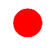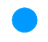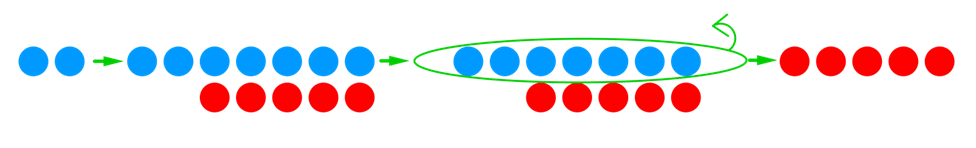# Introduction to integer subtraction#### All You Need in One Place

Everything you need for better marks in primary, GCSE, and A-level classes.#### Learn with Confidence

We’ve mastered the UK’s national curriculum so you can study with confidence.#### Instant and Unlimited Help

0/14
##### Examples
###### Lessons
1. What subtraction statement does each of the following diagrams represent?
+1:-1:1.2.3.4.2. Find the two differences between each pair of integers.
1. +2, + 9
2. +1, -5
3. -3, -7
3. Use integer subtraction to find the difference for the following situations.
1. Bob had 10 chocolate bars and he ate 3 of them.
2. Jamie earned $20 yesterday and$18 today. How much more money did he earn yesterday than today?
3. Tom borrowed $8 from John last week. This week Tom gave John$5 back. How much did Tom still owe?
4. Find the missing values.
1. (+8) – (__) = +5
2. (__) – (+6) = – 2
3. (– 5) – (__) = – 1
4. (__) – (– 8) = +6
0%
##### Practice
###### Topic Notes
Some of us just want to make a difference in the world. Well, in this section, you will make a difference using mathematics! In this section, we will be subtracting negative and positive integers using subtraction statements. First, we will use diagrams to help us with our subtracting. Then, we will be given integers and asked to find the differences between them. Next, we will be given word problems and asked to solve the problems using subtraction statements. Finally, we will be given subtraction statements and asked to find the missing values in the statements.